# Quadratic Equations and Functions Chapter Exam

Exam Instructions:

Choose your answers to the questions and click 'Next' to see the next set of questions. You can skip questions if you would like and come back to them later with the yellow "Go To First Skipped Question" button. When you have completed the practice exam, a green submit button will appear. Click it to see your results. Good luck!

### Page 1

#### Question 1 1. Which of the following are the solutions to the equation below?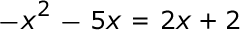#### Question 4 4. Rewrite the quadratic below in standard form: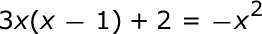### Page 2

#### Question 8 8. What is the b-value of the quadratic equation below?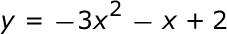#### Question 9 9. In order to complete the square on the following equation, what value would you add to both sides?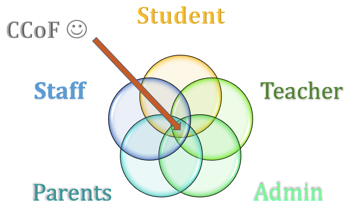### Page 3

#### Question 11 11. Find the roots of the equation.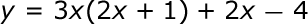#### Question 12 12. Expressing the equation below in the vertex form will give a value of 'h' = _____.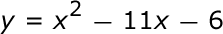#### Question 13 13. Solve the following equation.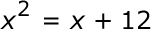#### Question 14 14. Use the quadratic formula to find the solutions to the equation below.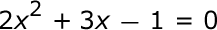### Page 4

#### Question 16 16. When completing the square for the quadratic equation shown below, how is c calculated?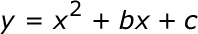#### Question 19 19. Find the roots of the equation below.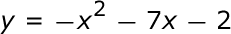#### Question 20 20. Find the solution to the equation below.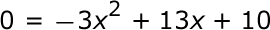### Page 5

#### Question 21 21. Find the value for c that must be added to both sides, and rewrite the equation in the vertex form.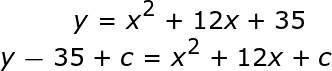#### Question 24 24. Find the solution to the equation below.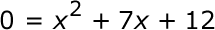### Page 6

#### Question 27 27. What would the first step be in completing the square of the following equation?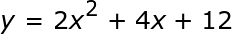#### Question 28 28. If the equation below told you a ball was 'h' feet in the air after 't' seconds, what would be the first step to finding out when it hit the ground?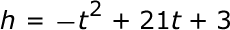#### Question 29 29. Rewrite the following equation in vertex form.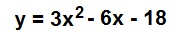#### Quadratic Equations and Functions Chapter Exam Instructions

Choose your answers to the questions and click 'Next' to see the next set of questions. You can skip questions if you would like and come back to them later with the yellow "Go To First Skipped Question" button. When you have completed the practice exam, a green submit button will appear. Click it to see your results. Good luck!

Support